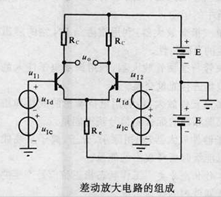# 差动放大器的基本概念

## 差动放大器的基本概念

2016-11-02 作者: xuzhiping 浏览: 2974 次

### 差动放大器的组成### 经典差动放大器$$V_{OUT}=( \frac {R4}{R3+R4} ) \times ( \frac {R1+R2}{R1} ) \times V2 - \frac {R2}{R1} V1$$

$$V_{OUT}=( \frac {R2}{R1} ) (V2-V1)$$

CMRR

$$CMRR≈ \frac {Ad+1}{4t}$$